# Nonparametric and Distribution- Free Statistics _contd

1. Aug 2012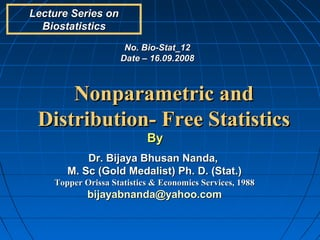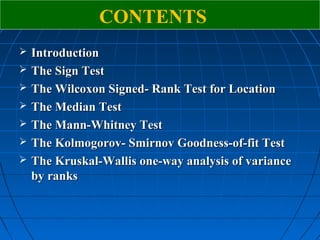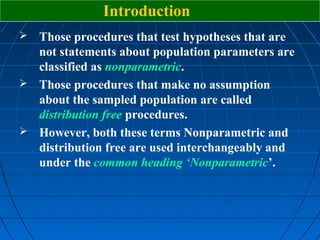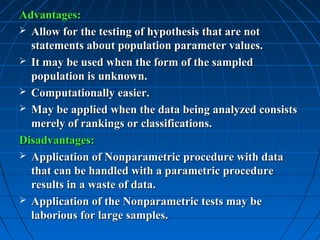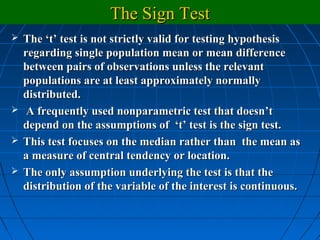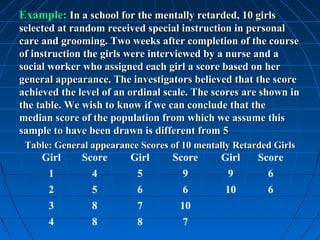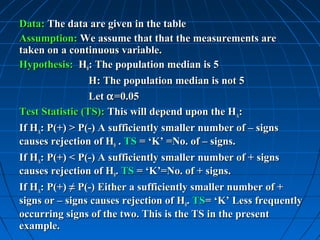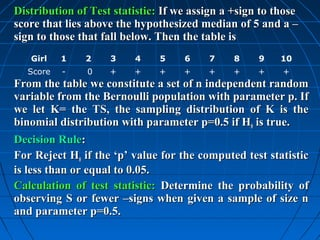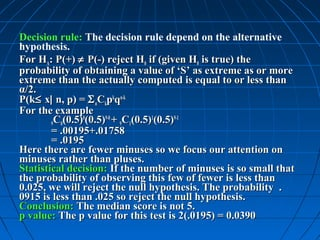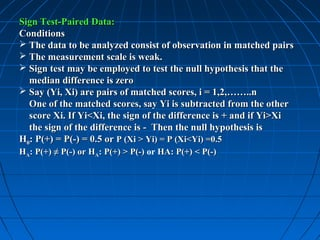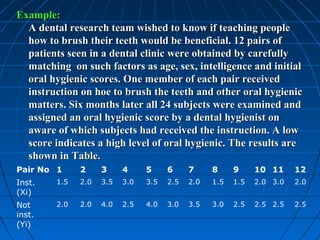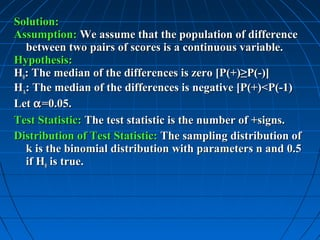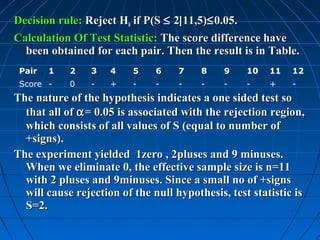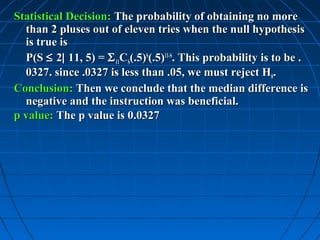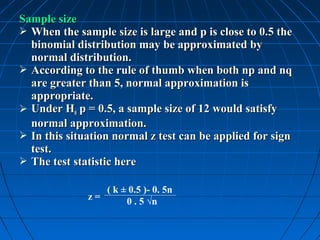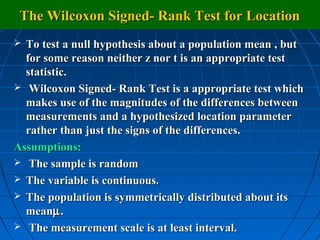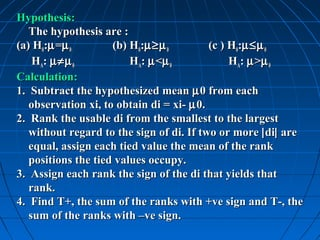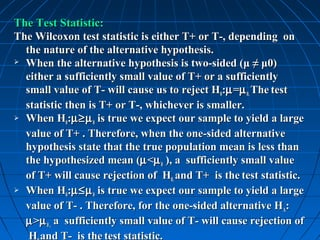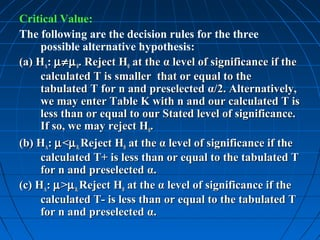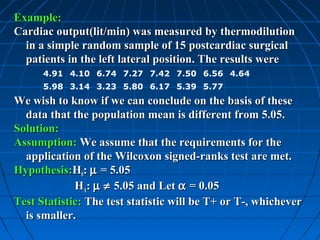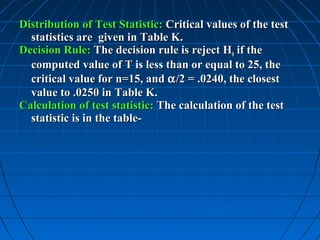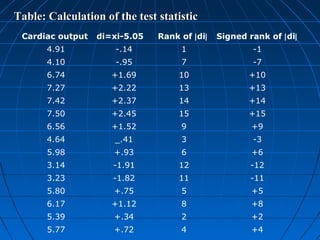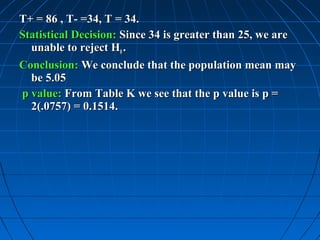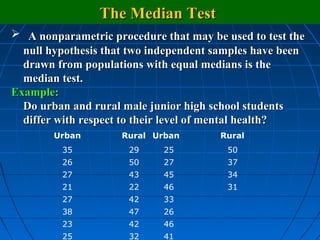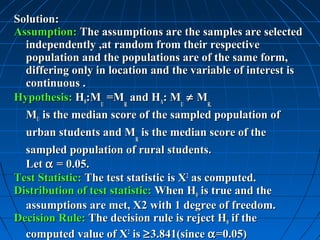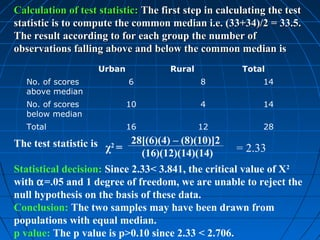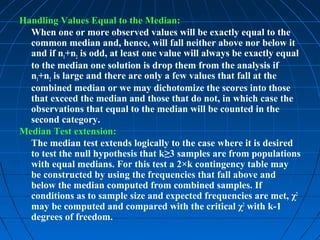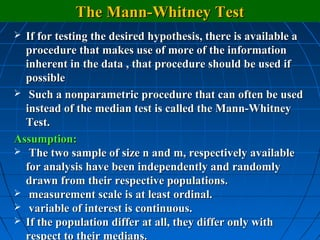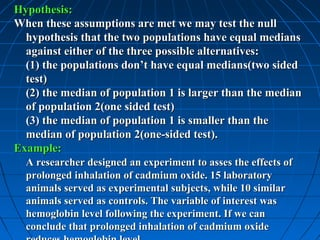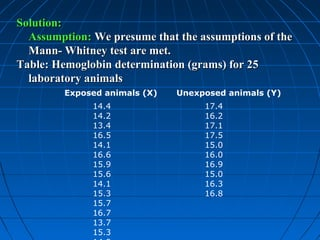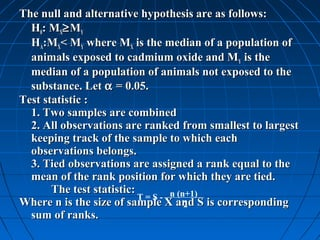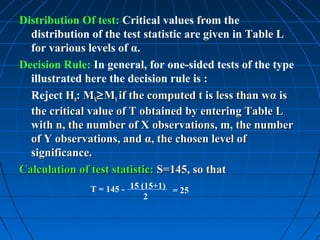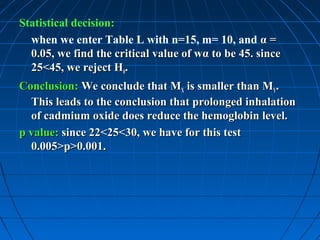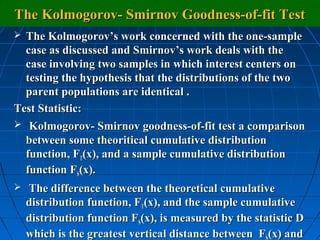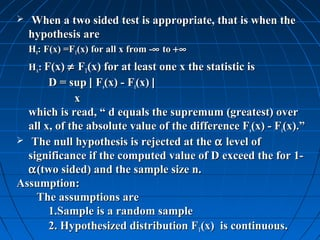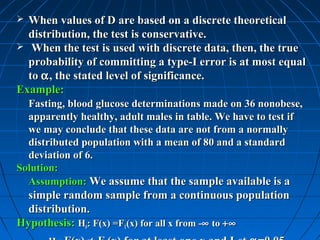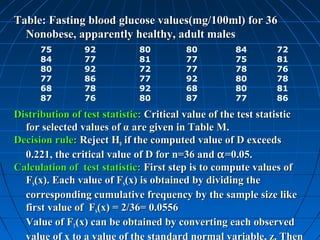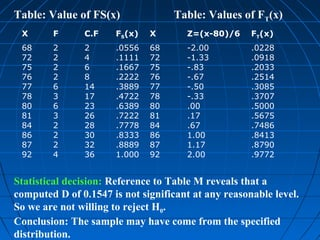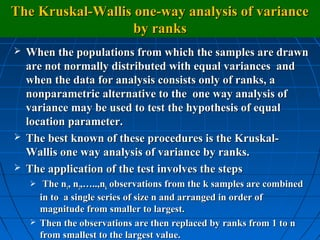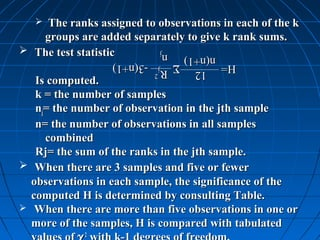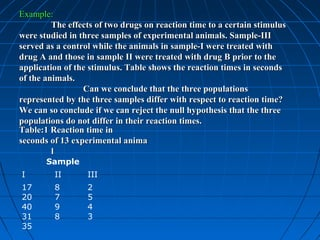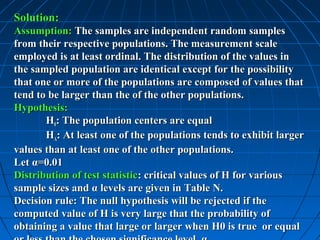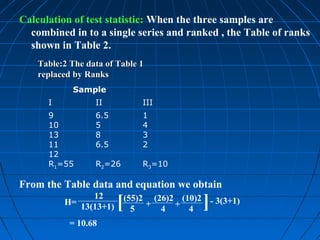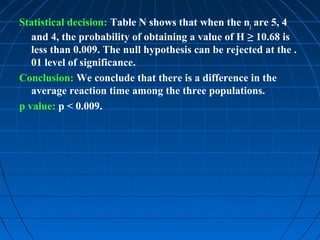1 von 44

### Nonparametric and Distribution- Free Statistics _contd

• 1. Lecture Series on Biostatistics No. Bio-Stat_12 Date – 16.09.2008 Nonparametric and Distribution- Free Statistics By Dr. Bijaya Bhusan Nanda, M. Sc (Gold Medalist) Ph. D. (Stat.) Topper Orissa Statistics & Economics Services, 1988 bijayabnanda@yahoo.com
• 2. CONTENTS  Introduction  The Sign Test  The Wilcoxon Signed- Rank Test for Location  The Median Test  The Mann-Whitney Test  The Kolmogorov- Smirnov Goodness-of-fit Test  The Kruskal-Wallis one-way analysis of variance by ranks
• 3. Introduction  Those procedures that test hypotheses that are not statements about population parameters are classified as nonparametric.  Those procedures that make no assumption about the sampled population are called distribution free procedures.  However, both these terms Nonparametric and distribution free are used interchangeably and under the common heading ‘Nonparametric’.
• 4. Advantages:  Allow for the testing of hypothesis that are not statements about population parameter values.  It may be used when the form of the sampled population is unknown.  Computationally easier.  May be applied when the data being analyzed consists merely of rankings or classifications. Disadvantages:  Application of Nonparametric procedure with data that can be handled with a parametric procedure results in a waste of data.  Application of the Nonparametric tests may be laborious for large samples.
• 5. The Sign Test  The ‘t’ test is not strictly valid for testing hypothesis regarding single population mean or mean difference between pairs of observations unless the relevant populations are at least approximately normally distributed.  A frequently used nonparametric test that doesn’t depend on the assumptions of ‘t’ test is the sign test.  This test focuses on the median rather than the mean as a measure of central tendency or location.  The only assumption underlying the test is that the distribution of the variable of the interest is continuous.
• 6. Example: In a school for the mentally retarded, 10 girls selected at random received special instruction in personal care and grooming. Two weeks after completion of the course of instruction the girls were interviewed by a nurse and a social worker who assigned each girl a score based on her general appearance. The investigators believed that the score achieved the level of an ordinal scale. The scores are shown in the table. We wish to know if we can conclude that the median score of the population from which we assume this sample to have been drawn is different from 5 Table: General appearance Scores of 10 mentally Retarded Girls Girl Score Girl Score Girl Score 1 4 5 9 9 6 2 5 6 6 10 6 3 8 7 10 4 8 8 7
• 7. Data: The data are given in the table Assumption: We assume that that the measurements are taken on a continuous variable. Hypothesis: H0: The population median is 5 H: The population median is not 5 Let α =0.05 Test Statistic (TS): This will depend upon the HA: If HA: P(+) > P(-) A sufficiently smaller number of – signs causes rejection of H0 . TS = ‘K’ =No. of – signs. If HA: P(+) < P(-) A sufficiently smaller number of + signs causes rejection of H0. TS = ‘K’=No. of + signs. If HA: P(+) ≠ P(-) Either a sufficiently smaller number of + signs or – signs causes rejection of H0. TS= ‘K’ Less frequently occurring signs of the two. This is the TS in the present example.
• 8. Distribution of Test statistic: If we assign a +sign to those score that lies above the hypothesized median of 5 and a – sign to those that fall below. Then the table is Girl 1 2 3 4 5 6 7 8 9 10 Score - 0 + + + + + + + + From the table we constitute a set of n independent random variable from the Bernoulli population with parameter p. If we let K= the TS, the sampling distribution of K is the binomial distribution with parameter p=0.5 if H0 is true. Decision Rule: For Reject H0 if the ‘p’ value for the computed test statistic is less than or equal to 0.05. Calculation of test statistic: Determine the probability of observing S or fewer –signs when given a sample of size n and parameter p=0.5.
• 9. Decision rule: The decision rule depend on the alternative hypothesis. For HA: P(+) ≠ P(-) reject H0 if (given H0 is true) the probability of obtaining a value of ‘S’ as extreme as or more extreme than the actually computed is equal to or less than α/2. P(k≤ x| n, p) = Σ nCkpkqn-k For the example 9C0(0.5) (0.5) + 9C1(0.5) (0.5) 0 9-0 1 9-1 = .00195+.01758 = .0195 Here there are fewer minuses so we focus our attention on minuses rather than pluses. Statistical decision: If the number of minuses is so small that the probability of observing this few of fewer is less than 0.025, we will reject the null hypothesis. The probability . 0915 is less than .025 so reject the null hypothesis. Conclusion: The median score is not 5. p value: The p value for this test is 2(.0195) = 0.0390
• 10. Sign Test-Paired Data: Conditions  The data to be analyzed consist of observation in matched pairs  The measurement scale is weak.  Sign test may be employed to test the null hypothesis that the median difference is zero  Say (Yi, Xi) are pairs of matched scores, i = 1,2,……..n One of the matched scores, say Yi is subtracted from the other score Xi. If Yi<Xi, the sign of the difference is + and if Yi>Xi the sign of the difference is - Then the null hypothesis is H0: P(+) = P(-) = 0.5 or P (Xi > Yi) = P (Xi<Yi) =0.5 HA: P(+) ≠ P(-) or HA: P(+) > P(-) or HA: P(+) < P(-)
• 11. Example: A dental research team wished to know if teaching people how to brush their teeth would be beneficial. 12 pairs of patients seen in a dental clinic were obtained by carefully matching on such factors as age, sex, intelligence and initial oral hygienic scores. One member of each pair received instruction on hoe to brush the teeth and other oral hygienic matters. Six months later all 24 subjects were examined and assigned an oral hygienic score by a dental hygienist on aware of which subjects had received the instruction. A low score indicates a high level of oral hygienic. The results are shown in Table. Pair No 1 2 3 4 5 6 7 8 9 10 11 12 Inst. 1.5 2.0 3.5 3.0 3.5 2.5 2.0 1.5 1.5 2.0 3.0 2.0 (Xi) Not 2.0 2.0 4.0 2.5 4.0 3.0 3.5 3.0 2.5 2.5 2.5 2.5 inst. (Yi)
• 12. Solution: Assumption: We assume that the population of difference between two pairs of scores is a continuous variable. Hypothesis: H0: The median of the differences is zero [P(+)≥P(-)] HA: The median of the differences is negative [P(+)<P(-1) Let α =0.05. Test Statistic: The test statistic is the number of +signs. Distribution of Test Statistic: The sampling distribution of k is the binomial distribution with parameters n and 0.5 if H0 is true.
• 13. Decision rule: Reject H0 if P(S ≤ 2| 11,5)≤ 0.05. Calculation Of Test Statistic: The score difference have been obtained for each pair. Then the result is in Table. Pair 1 2 3 4 5 6 7 8 9 10 11 12 Score - 0 - + - - - - - - + - The nature of the hypothesis indicates a one sided test so that all of α = 0.05 is associated with the rejection region, which consists of all values of S (equal to number of +signs). The experiment yielded 1zero , 2pluses and 9 minuses. When we eliminate 0, the effective sample size is n=11 with 2 pluses and 9minuses. Since a small no of +signs will cause rejection of the null hypothesis, test statistic is S=2.
• 14. Statistical Decision: The probability of obtaining no more than 2 pluses out of eleven tries when the null hypothesis is true is P(S ≤ 2| 11, 5) = Σ 11CS(.5)S(.5)11-S. This probability is to be . 0327. since .0327 is less than .05, we must reject H0. Conclusion: Then we conclude that the median difference is negative and the instruction was beneficial. p value: The p value is 0.0327
• 15. Sample size  When the sample size is large and p is close to 0.5 the binomial distribution may be approximated by normal distribution.  According to the rule of thumb when both np and nq are greater than 5, normal approximation is appropriate.  Under H0 p = 0.5, a sample size of 12 would satisfy normal approximation.  In this situation normal z test can be applied for sign test.  The test statistic here ( k ± 0.5 )- 0. 5n z= 0 . 5 √n
• 16. The Wilcoxon Signed- Rank Test for Location  To test a null hypothesis about a population mean , but for some reason neither z nor t is an appropriate test statistic.  Wilcoxon Signed- Rank Test is a appropriate test which makes use of the magnitudes of the differences between measurements and a hypothesized location parameter rather than just the signs of the differences. Assumptions:  The sample is random  The variable is continuous.  The population is symmetrically distributed about its meanµ .  The measurement scale is at least interval.
• 17. Hypothesis: The hypothesis are : (a) H0:µ =µ 0 (b) H0:µ≥µ 0 (c ) H0:µ≤µ 0 HA: µ≠µ 0 HA: µ <µ 0 H A: µ > µ 0 Calculation: 1. Subtract the hypothesized mean µ 0 from each observation xi, to obtain di = xi- µ 0. 2. Rank the usable di from the smallest to the largest without regard to the sign of di. If two or more | di| are equal, assign each tied value the mean of the rank positions the tied values occupy. 3. Assign each rank the sign of the di that yields that rank. 4. Find T+, the sum of the ranks with +ve sign and T-, the sum of the ranks with –ve sign.
• 18. The Test Statistic: The Wilcoxon test statistic is either T+ or T-, depending on the nature of the alternative hypothesis.  When the alternative hypothesis is two-sided (μ ≠ μ0) either a sufficiently small value of T+ or a sufficiently small value of T- will cause us to reject H0:µ =µ 0. The test statistic then is T+ or T-, whichever is smaller.  When H :µ≥µ is true we expect our sample to yield a large 0 0 value of T+ . Therefore, when the one-sided alternative hypothesis state that the true population mean is less than the hypothesized mean (µ <µ 0 ), a sufficiently small value of T+ will cause rejection of H0. and T+ is the test statistic.  When H :µ≤µ is true we expect our sample to yield a large 0 0 value of T- . Therefore, for the one-sided alternative HA: µ >µ 0 , a sufficiently small value of T- will cause rejection of H and T- is the test statistic.
• 19. Critical Value: The following are the decision rules for the three possible alternative hypothesis: (a) HA: µ≠µ 0. Reject H0 at the α level of significance if the calculated T is smaller that or equal to the tabulated T for n and preselected α/2. Alternatively, we may enter Table K with n and our calculated T is less than or equal to our Stated level of significance. If so, we may reject H0. (b) HA: µ <µ 0. Reject H0 at the α level of significance if the calculated T+ is less than or equal to the tabulated T for n and preselected α. (c) HA: µ >µ 0. Reject H0 at the α level of significance if the calculated T- is less than or equal to the tabulated T for n and preselected α.
• 20. Example: Cardiac output(lit/min) was measured by thermodilution in a simple random sample of 15 postcardiac surgical patients in the left lateral position. The results were 4.91 4.10 6.74 7.27 7.42 7.50 6.56 4.64 5.98 3.14 3.23 5.80 6.17 5.39 5.77 We wish to know if we can conclude on the basis of these data that the population mean is different from 5.05. Solution: Assumption: We assume that the requirements for the application of the Wilcoxon signed-ranks test are met. Hypothesis:H0: µ = 5.05 HA: µ ≠ 5.05 and Let α = 0.05 Test Statistic: The test statistic will be T+ or T-, whichever is smaller.
• 21. Distribution of Test Statistic: Critical values of the test statistics are given in Table K. Decision Rule: The decision rule is reject H0 if the computed value of T is less than or equal to 25, the critical value for n=15, and α /2 = .0240, the closest value to .0250 in Table K. Calculation of test statistic: The calculation of the test statistic is in the table-
• 22. Table: Calculation of the test statistic Cardiac output di=xi-5.05 Rank of |di| Signed rank of |di| 4.91 -.14 1 -1 4.10 -.95 7 -7 6.74 +1.69 10 +10 7.27 +2.22 13 +13 7.42 +2.37 14 +14 7.50 +2.45 15 +15 6.56 +1.52 9 +9 4.64 _.41 3 -3 5.98 +.93 6 +6 3.14 -1.91 12 -12 3.23 -1.82 11 -11 5.80 +.75 5 +5 6.17 +1.12 8 +8 5.39 +.34 2 +2 5.77 +.72 4 +4
• 23. T+ = 86 , T- =34, T = 34. Statistical Decision: Since 34 is greater than 25, we are unable to reject H0 . Conclusion: We conclude that the population mean may be 5.05 p value: From Table K we see that the p value is p = 2(.0757) = 0.1514.
• 24. The Median Test  A nonparametric procedure that may be used to test the null hypothesis that two independent samples have been drawn from populations with equal medians is the median test. Example: Do urban and rural male junior high school students differ with respect to their level of mental health? Urban Rural Urban Rural 35 29 25 50 26 50 27 37 27 43 45 34 21 22 46 31 27 42 33 38 47 26 23 42 46 25 32 41
• 25. Solution: Assumption: The assumptions are the samples are selected independently ,at random from their respective population and the populations are of the same form, differing only in location and the variable of interest is continuous . Hypothesis: H0:MU =MR and HA: MU ≠ MR. MU is the median score of the sampled population of urban students and MR is the median score of the sampled population of rural students. Let α = 0.05. Test Statistic: The test statistic is X2 as computed. Distribution of test statistic: When H0 is true and the assumptions are met, X2 with 1 degree of freedom. Decision Rule: The decision rule is reject H0 if the computed value of X2 is ≥ 3.841(since α =0.05)
• 26. Calculation of test statistic: The first step in calculating the test statistic is to compute the common median i.e. (33+34)/2 = 33.5. The result according to for each group the number of observations falling above and below the common median is Urban Rural Total No. of scores 6 8 14 above median No. of scores 10 4 14 below median Total 16 12 28 The test statistic is χ2 = 28[(6)(4) – (8)(10)]2 = 2.33 (16)(12)(14)(14) Statistical decision: Since 2.33< 3.841, the critical value of X2 with α=.05 and 1 degree of freedom, we are unable to reject the null hypothesis on the basis of these data. Conclusion: The two samples may have been drawn from populations with equal median. p value: The p value is p>0.10 since 2.33 < 2.706.
• 27. Handling Values Equal to the Median: When one or more observed values will be exactly equal to the common median and, hence, will fall neither above nor below it and if n1+n2 is odd, at least one value will always be exactly equal to the median one solution is drop them from the analysis if n1+n2 is large and there are only a few values that fall at the combined median or we may dichotomize the scores into those that exceed the median and those that do not, in which case the observations that equal to the median will be counted in the second category. Median Test extension: The median test extends logically to the case where it is desired to test the null hypothesis that k≥3 samples are from populations with equal medians. For this test a 2×k contingency table may be constructed by using the frequencies that fall above and below the median computed from combined samples. If conditions as to sample size and expected frequencies are met, χ2 may be computed and compared with the critical χ2 with k-1 degrees of freedom.
• 28. The Mann-Whitney Test  If for testing the desired hypothesis, there is available a procedure that makes use of more of the information inherent in the data , that procedure should be used if possible  Such a nonparametric procedure that can often be used instead of the median test is called the Mann-Whitney Test. Assumption:  The two sample of size n and m, respectively available for analysis have been independently and randomly drawn from their respective populations.  measurement scale is at least ordinal.  variable of interest is continuous.  If the population differ at all, they differ only with respect to their medians.
• 29. Hypothesis: When these assumptions are met we may test the null hypothesis that the two populations have equal medians against either of the three possible alternatives: (1) the populations don’t have equal medians(two sided test) (2) the median of population 1 is larger than the median of population 2(one sided test) (3) the median of population 1 is smaller than the median of population 2(one-sided test). Example: A researcher designed an experiment to asses the effects of prolonged inhalation of cadmium oxide. 15 laboratory animals served as experimental subjects, while 10 similar animals served as controls. The variable of interest was hemoglobin level following the experiment. If we can conclude that prolonged inhalation of cadmium oxide
• 30. Solution: Assumption: We presume that the assumptions of the Mann- Whitney test are met. Table: Hemoglobin determination (grams) for 25 laboratory animals Exposed animals (X) Unexposed animals (Y) 14.4 17.4 14.2 16.2 13.4 17.1 16.5 17.5 14.1 15.0 16.6 16.0 15.9 16.9 15.6 15.0 14.1 16.3 15.3 16.8 15.7 16.7 13.7 15.3
• 31. The null and alternative hypothesis are as follows: H0: MX≥ MY HA:MX< MY where MX is the median of a population of animals exposed to cadmium oxide and MY is the median of a population of animals not exposed to the substance. Let α = 0.05. Test statistic : 1. Two samples are combined 2. All observations are ranked from smallest to largest keeping track of the sample to which each observations belongs. 3. Tied observations are assigned a rank equal to the mean of the rank position for which they are tied. The test statistic: T = S - n (n+1) Where n is the size of sample X and S is corresponding 2 sum of ranks.
• 32. Distribution Of test: Critical values from the distribution of the test statistic are given in Table L for various levels of α. Decision Rule: In general, for one-sided tests of the type illustrated here the decision rule is : Reject H0: MX≥ MY if the computed t is less than wα is the critical value of T obtained by entering Table L with n, the number of X observations, m, the number of Y observations, and α, the chosen level of significance. Calculation of test statistic: S=145, so that T = 145 - 15 (15+1) = 25 2
• 33. Statistical decision: when we enter Table L with n=15, m= 10, and α = 0.05, we find the critical value of wα to be 45. since 25<45, we reject H0. Conclusion: We conclude that MX is smaller than MY. This leads to the conclusion that prolonged inhalation of cadmium oxide does reduce the hemoglobin level. p value: since 22<25<30, we have for this test 0.005>p>0.001.
• 34. The Kolmogorov- Smirnov Goodness-of-fit Test  The Kolmogorov’s work concerned with the one-sample case as discussed and Smirnov’s work deals with the case involving two samples in which interest centers on testing the hypothesis that the distributions of the two parent populations are identical . Test Statistic:  Kolmogorov- Smirnov goodness-of-fit test a comparison between some theoritical cumulative distribution function, FT(x), and a sample cumulative distribution function FS(x).  The difference between the theoretical cumulative distribution function, FT(x), and the sample cumulative distribution function FS(x), is measured by the statistic D which is the greatest vertical distance between F (x) and
• 35. When a two sided test is appropriate, that is when the hypothesis are H0: F(x) =FT(x) for all x from -∞ to +∞ HA: F(x) ≠ FT(x) for at least one x the statistic is D = sup | Fs(x) - Ft(x) | x which is read, “ d equals the supremum (greatest) over all x, of the absolute value of the difference Fs(x) - Ft(x).”  The null hypothesis is rejected at the α level of significance if the computed value of D exceed the for 1- α (two sided) and the sample size n. Assumption: The assumptions are 1.Sample is a random sample 2. Hypothesized distribution FT(x) is continuous.
• 36.  When values of D are based on a discrete theoretical distribution, the test is conservative.  When the test is used with discrete data, then, the true probability of committing a type-I error is at most equal to α , the stated level of significance. Example: Fasting, blood glucose determinations made on 36 nonobese, apparently healthy, adult males in table. We have to test if we may conclude that these data are not from a normally distributed population with a mean of 80 and a standard deviation of 6. Solution: Assumption: We assume that the sample available is a simple random sample from a continuous population distribution. Hypothesis: H0: F(x) =FT(x) for all x from -∞ to +∞
• 37. Table: Fasting blood glucose values(mg/100ml) for 36 Nonobese, apparently healthy, adult males 75 92 80 80 84 72 84 77 81 77 75 81 80 92 72 77 78 76 77 86 77 92 80 78 68 78 92 68 80 81 87 76 80 87 77 86 Distribution of test statistic: Critical value of the test statistic for selected values of α are given in Table M. Decision rule: Reject H0 if the computed value of D exceeds 0.221, the critical value of D for n=36 and α =0.05. Calculation of test statistic: First step is to compute values of FS(x). Each value of FS(x) is obtained by dividing the corresponding cumulative frequency by the sample size like first value of FS(x) = 2/36= 0.0556 Value of FT(x) can be obtained by converting each observed value of x to a value of the standard normal variable, z. Then
• 38. Table: Value of FS(x) Table: Values of FT(x) X F C.F FS(x) X Z=(x-80)/6 FT(x) 68 2 2 .0556 68 -2.00 .0228 72 2 4 .1111 72 -1.33 .0918 75 2 6 .1667 75 -.83 .2033 76 2 8 .2222 76 -.67 .2514 77 6 14 .3889 77 -.50 .3085 78 3 17 .4722 78 -.33 .3707 80 6 23 .6389 80 .00 .5000 81 3 26 .7222 81 .17 .5675 84 2 28 .7778 84 .67 .7486 86 2 30 .8333 86 1.00 .8413 87 2 32 .8889 87 1.17 .8790 92 4 36 1.000 92 2.00 .9772 Statistical decision: Reference to Table M reveals that a computed D of 0.1547 is not significant at any reasonable level. So we are not willing to reject H0. Conclusion: The sample may have come from the specified distribution.
• 39. The Kruskal-Wallis one-way analysis of variance by ranks  When the populations from which the samples are drawn are not normally distributed with equal variances and when the data for analysis consists only of ranks, a nonparametric alternative to the one way analysis of variance may be used to test the hypothesis of equal location parameter.  The best known of these procedures is the Kruskal- Wallis one way analysis of variance by ranks.  The application of the test involves the steps  The n1, n2,…..,nk observations from the k samples are combined in to a single series of size n and arranged in order of magnitude from smaller to largest.  Then the observations are then replaced by ranks from 1 to n from smallest to the largest value.
• 40. The ranks assigned to observations in each of the k groups are added separately to give k rank sums.  The test statistic n(n+1) nj n(n+1) nj H= H= Σ R -3(n+1) Σ Rjj -3(n+1) 12 12 2 2 Is computed. k = the number of samples nj= the number of observation in the jth sample n= the number of observations in all samples combined Rj= the sum of the ranks in the jth sample.  When there are 3 samples and five or fewer observations in each sample, the significance of the computed H is determined by consulting Table.  When there are more than five observations in one or more of the samples, H is compared with tabulated 2
• 41. Example: The effects of two drugs on reaction time to a certain stimulus were studied in three samples of experimental animals. Sample-III served as a control while the animals in sample-I were treated with drug A and those in sample II were treated with drug B prior to the application of the stimulus. Table shows the reaction times in seconds of the animals. Can we conclude that the three populations represented by the three samples differ with respect to reaction time? We can so conclude if we can reject the null hypothesis that the three populations do not differ in their reaction times. Table:1 Reaction time in seconds of 13 experimental anima l Sample I II III 17 8 2 20 7 5 40 9 4 31 8 3 35
• 42. Solution: Assumption: The samples are independent random samples from their respective populations. The measurement scale employed is at least ordinal. The distribution of the values in the sampled population are identical except for the possibility that one or more of the populations are composed of values that tend to be larger than the of the other populations. Hypothesis: H0: The population centers are equal HA: At least one of the populations tends to exhibit larger values than at least one of the other populations. Let α=0.01 Distribution of test statistic: critical values of H for various sample sizes and α levels are given in Table N. Decision rule: The null hypothesis will be rejected if the computed value of H is very large that the probability of obtaining a value that large or larger when H0 is true or equal
• 43. Calculation of test statistic: When the three samples are combined in to a single series and ranked , the Table of ranks shown in Table 2. Table:2 The data of Table 1 replaced by Ranks Sample I II III 9 6.5 1 10 5 4 13 8 3 11 6.5 2 12 R1=55 R2=26 R3=10 From the Table data and equation we obtain H= 12 13(13+1) [(55)2 + (26)2 + (10)2 ] - 3(3+1) 5 4 4 = 10.68
• 44. Statistical decision: Table N shows that when the nj are 5, 4 and 4, the probability of obtaining a value of H ≥ 10.68 is less than 0.009. The null hypothesis can be rejected at the . 01 level of significance. Conclusion: We conclude that there is a difference in the average reaction time among the three populations. p value: p < 0.009.